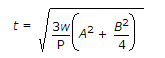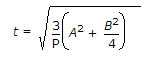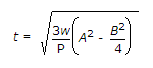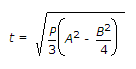# Civil Engineering - Steel Structure Design

### Exercise :: Steel Structure Design - Section 5

21.

The minimum width B of a solid casing for a cased beam, is equal to

 A. B = b + 25 mm B. B = b + 50 mm C. B = b + 75 mm D. B = b + 100 mm E. B = b + 125 mm

Explanation:

No answer description available for this question. Let us discuss.

22.

Design of a riveted joint is based on the assumption:

 A. Bending stress in rivets is accounted for B. Riveted hole is assumed to be completely filled by the rivet C. Stress in the plate in not uniform D. Friction between plates is taken into account E. None of these.

Explanation:

No answer description available for this question. Let us discuss.

23.

A web plate is called unstiffened if the ratio of clear depth to thickness is less than

 A. 35 B. 50 C. 60 D. 85 E. 90

Explanation:

No answer description available for this question. Let us discuss.

24.

Pick up the correct statement from the following:

 A. The slenderness ratio of lacing bars for compression members should not exceed 145 B. The minimum width of lacing bar connected with rivets of nominal diameter 16 mm, is kept 50 mm C. The minimum thickness of a flat lacing bar is kept equal to one-fortieth of its length between inner end rivets D. The angle of inclination of lacing bars and the axis of the members should be between 40° and 70° E. All the above.

Explanation:

No answer description available for this question. Let us discuss.

25.

If P is the allowable bending stress in a slab, whose greater and lesser projections from the column faces, are A and B, the thickness (t) of the slab base, is (where w is the intensity of earth pressure.)

 A.B.C.D.